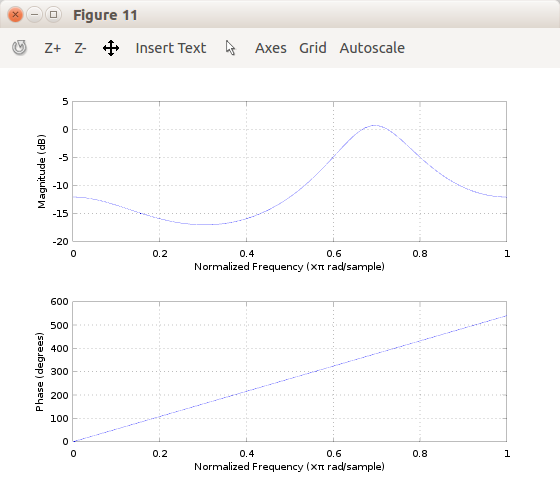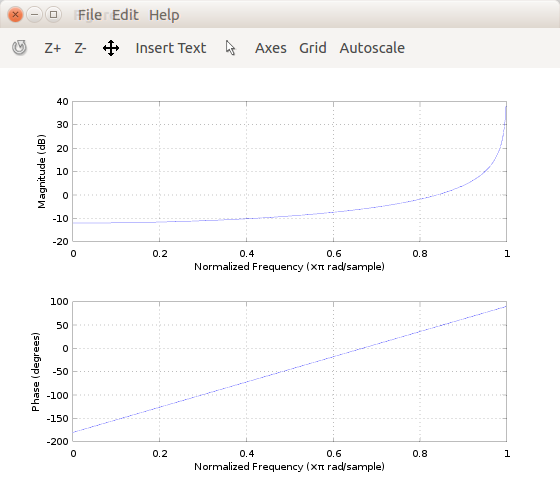## Parallel and series connection, what difference it makesStarted by 6 years ago4 replieslatest reply 6 years ago86 views

Hi!

Lets assume you have two 1st order IIR filters:

    [b1 b2 a1 a2]
1 = [ 1 -1 -1 -1]
2 = [-1 -1 -1  1]

What difference in phase and magnitude responses it makes when you use these filters

1. parallelly
2. in series

?

Meaning does responses become summed, subtracted, etc..

EDIT: Here are the two filters plotted:[ - ]Without math I will use quick matalb commands:

a1 = [1 -1 -1 -1];
a2 = [-1 -1 -1 1];

[ - ]Your commands give these results in Octave:Parallel:When those two filters are used either parallelly or in series, shouldn't I be able to hear the -10dB - -15dB magnitude change showing in plots ... but, I don't hear it (actually, series connection does not change gain, parallel connection boosts gain) so, is what you suggested the right method to measure the differences I'm talking about?

[ - ]according to rules of filter function you need to add initial 1 for IIR as below:

I also realised you are using b,a possibly meaning forward/reverse coeff

b1 = [1 -1];

b2 = [-1 -1];

a1 = [1 -1 -1];
a2 = [1 -1 1];

As to your question you need to explain what is your input and how you observe output and what filters you use

[ - ]% 1st order all pass filter% H(s) = (s/w0 - 1) / (s/w0 + 1)
w0 = 2 * pi * f; % Hz to rad/sb = [w0 -1];a = [w0 1];